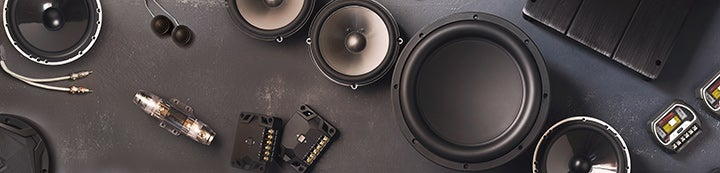1 - 7 of 7 Posts

·
##### Registered
Joined
·
137 Posts
Discussion Starter · ·
If I were parallel the wires from an 8ohm 6.5inch mid with a 4ohm 4inch. What would be the final ohm load? Any ideas.

#### MiniVanMan

·
##### Registered
Joined
·
2,298 Posts
Technically 6 ohms. But only for the frequencies where the 2 speakers intersect. In other words if your 6.5" is playing the frequencies 80-2000 hz and your 4" is playing 150-4000 hz, the amplifier will see an 8 ohm load for frequencies 80-150 hz, a 6 ohm load for frequencies 150-2000 hz and a 4 ohm load for 2000-4000 hz.

Clear as mud??? Good.

#### AzGrower

·
##### Registered
Joined
·
2,410 Posts
In theory it would be 6 ohms, but I dont think that is possible. But I am probably wrong on both questions...

·
##### Registered
Joined
·
17,148 Posts
2.66666666 ohms

Final impedance= (R1XR2)/(R1+R2)
Down and dirty for calculating 2 impedances

Many drivers=(1/R1)+(1/R2)+(1/R3)+........

So for example if you have 2X4 ohm drivers and 1X8 ohm

You could do the first calculation twice;
(4X4)/(4+4)=2 ohms
Then
(2X8)/(2+8)=1.6 ohm final

lotta math

2nd method

(1/4)+(1/4)+(1/8)+=0.625
1/.0625= 1.6

the 2nd method is easier with a normal calculator using the memory function

Keystrokes are as follows
1 / 4 = M+ M+ (there's 2 4 ohm in the calc no sense in doing it twice)
1 / 8 = M+
1 / Mr

Bingo!

Hope this helps!

·
##### Registered
Joined
·
17,148 Posts
The final impedance of a speaker load will ALWAYS be less athan the lowest impedance in the formula.

It's a load if you take a load and put a lighter load on top of it then it does not get lighter.

But, as already mentioned this is only true if the drivers are playing in the same passband, and remember it's impedance not resistance. These are nominal numbers and impedance curves can widely vary from driver to driver.

#### AzGrower

·
##### Registered
Joined
·
2,410 Posts
Dooh, I knew I was wrong, I just took 4 plus 2 to get 6.

#### MiniVanMan

·
##### Registered
Joined
·
2,298 Posts
I guess it really was "clear as mud". That answers that for all times. Thanks for clearing that up for me also.

1 - 7 of 7 Posts